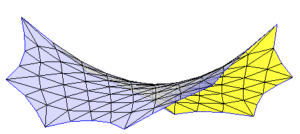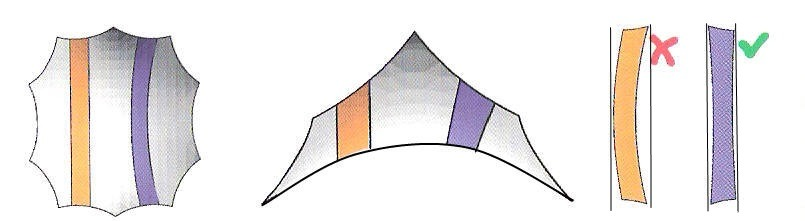In the section of general introduction to WinTess3, we have mentioned about geodesic lines. Why do we use geodesic lines?We could explain the reason why we use this type of lines in generating a pattern. However there are times that a picture (an example, in this case) is worth of a thousand words.

Suppose that we have a membrane like the image on the left. It’s a membrane obtained from a regular decagon with two opposite vertices raised to a certain value and the other adjacent vertices to a smaller value.

For this membrane, we want to design the pattern and decided to do it in direction of the highest points. We set the width of pattern according to the width of the membrane fabric we use. We draw a few lines on the surface of the membrane to obtain the pattern. How do we draw these lines?

We’re going to assume that there are two ways:

1. Lines that are straight on plane view (as corresponds to the orange pattern of the figure below).
2. Lines that look like as if they are radial (shown as the violet pattern of the figure below)

The two patterns use the same start and end points (the membrane is symmetrical).In the image on the right of the above figure, we see two patterns type, made by method 1 (orange) and method 2 (violet).

We clearly see that for a pattern of the same effective width, we need a roll of material with much wider than the second. In addition the amount of wasted material is also much higher in the first case than in the second.

Well, in fact the lines that make up the second pattern are geodesic lines. Let’s see how to draw them.